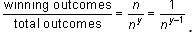Courses

# Test: Probability- 3

## 15 Questions MCQ Test Practice Questions for GMAT | Test: Probability- 3

Description
This mock test of Test: Probability- 3 for GMAT helps you for every GMAT entrance exam. This contains 15 Multiple Choice Questions for GMAT Test: Probability- 3 (mcq) to study with solutions a complete question bank. The solved questions answers in this Test: Probability- 3 quiz give you a good mix of easy questions and tough questions. GMAT students definitely take this Test: Probability- 3 exercise for a better result in the exam. You can find other Test: Probability- 3 extra questions, long questions & short questions for GMAT on EduRev as well by searching above.
QUESTION: 1

### A fair coin is flipped three times. What is the probability that the coin lands on heads exactly twice?

Solution:

There are 2 possible outcomes on each flip: heads or tails. Since the coin is flipped three times, there are 2 × 2 × 2 = 8 total possibilities: HHH, HHT, HTH, HTT, TTT, TTH, THT, THH.
Of these 8 possibilities, how many involve exactly two heads? We can simply count these up: HHT, HTH, THH. We see that there are 3 outcomes that involve exactly two heads. Thus, the correct answer is 3/8.
Alternatively, we can draw an anagram table to calculate the number of outcomes that involve exactly 2 heads.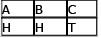The top row of the anagram table represents the 3 coin flips: A, B, and C. The bottom row of the anagram table represents one possible way to achieve the desired outcome of exactly two heads. The top row of the anagram yields 3!, which must be divided by 2! since the bottom row of the anagram table contains 2 repetitions of the letter H.
There are 3!/2! = 3 different outcomes that contain exactly 2 heads.
The probability of the coin landing on heads exactly twice is (# of two-head results) ÷ (total # of outcomes) = 3/8.

QUESTION: 2

### There are 10 women and 3 men in room A. One person is picked at random from room A and moved to room B, where there are already 3 women and 5 men. If a single person is then to be picked from room B, what is the probability that a woman will be picked?

Solution:

In order to solve this problem, we have to consider two different scenarios. In the first scenario, a woman is picked from room A and a woman is picked from room B. In the second scenario, a man is picked from room A and a woman is picked from room B. The probability that a woman is picked from room A is 10/13. If that woman is then added to room B, this means that there are 4 women and 5 men in room B (Originally there were 3 women and 5 men). So, the probability that a woman is picked from room B is 4/9.
Because we are calculating the probability of picking a woman from room A AND then from room B, we need to multiply these two probabilities: 10/13 x 4/9 = 40/117 The probability that a man is picked from room A is 3/13. If that man is then added to room B, this means that there are 3 women and 6 men in room B. So, the probability that a woman is picked from room B is 3/9.
Again, we multiply thse two probabilities: 3/13 x 3/9 = 9/117 To find the total probability that a woman will be picked from room B, we need to take both scenarios into account.
In other words, we need to consider the probability of picking a woman and a woman OR a man and a woman. In probabilities, OR means addition. If we add the two probabilities, we get: 40/117 + 9/117 = 49/117

QUESTION: 3

### If the probability of rain on any given day in Chicago during the summer i s 50%, independent of what happens on any other day, what is the probability of having exactly 3 rainy days from July 4 through July 8, inclusive?

Solution:

he period from July 4 to July 8, inclusive, contains 8 – 4 + 1 = 5 days, so we can rephrase the question as “What is the probability of having exactly 3 rainy days out of 5?” Since there are 2 possible outcomes for each day (R = rain or S = shine) and 5 days total, there are 2 x 2 x 2 x 2 x 2 = 32 possible scenarios for the 5 day period (RRRSS, RSRSS, SSRRR, etc…) To find the probability of having exactly three rainy days out of five, we must find the total number of scenarios containing exactly 3 R’s and 2 S’s, that is the number of possible RRRSS anagrams: = 5! / 2!3! = (5 x 4)/2 x 1 = 10 The probability then of having exactly 3 rainy days out of five is 10/32 or 5/16. Note that we were able to calculate the probability this way because the probability that any given scenario would occur was the same. This stemmed from the fact that the probability of rain = shine = 50%. Another way to solve this question would be to find the probability that one of the favorable scenarios would occur and to multiply that by the number of favorable scenarios. In this case, the probability that RRRSS (1st three days rain, last two shine) would occur is (1/2)(1/2)(1/2)(1/2)(1/2) = 1/32. There are 10 such scenarios (different anagrams of RRRSS) so the overall probability of exactly 3 rainy days out of 5 is again 10/32. This latter method works even when the likelihood of rain does not equal the likelihood of shine.

QUESTION: 4

In a shipment of 20 cars, 3 are found to be defective. If four car s are selected at random, what is the probability that exactly one of the four will be defective?

Solution:

There are four possible ways to pick exactly one defective car when picking four cars: DFFF, FDFF, FFDF, FFFD (D = defective, F = functional). To find the total probability we must find the probability of each one of these scenarios and add them together (we add because the total probability is the first scenario OR the second OR…). The probability of the first scenario is the probability of picking a defective car first (3/20) AND then a functional car (17/19) AND then another functional car (16/18) AND then another functional car (15/17). The probability of this first scenario is the product of these four probabilities: 3/20 x 17/19 x 16/18 x 15/17 = 2/19 The probability of each of the other three scenarios would also be 2/19 since the chance of getting the D first is the same as getting it second, third or fourth.
The total probability of getting exactly one defective car out of four = 2/19 + 2/19 + 2/19 + 2/19 = 8/19.

QUESTION: 5

A certain bag of gemstones is composed of two-thirds diamonds and one-third rubies. If the probability of randomly selecting two diamonds from the bag, without replacement, is 5/12, what is the probability of selecting two rubies from the bag, without replacement?

Solution:

The simplest way to solve the problem is to recognize that the total number of gems in the bag must be a multiple of 3, since we have 2/3 diamonds and 1/3 rubies. If we had a total number that was not divisible by 3, we would not be able to divide the stones into thirds. Given this fact, we can test some multiples of 3 to see whether any fit the description in the question. The smallest number of gems we could have is 6: 4 diamonds and 2 rubies (since we need at least 2 rubies). Is the probability of selecting two of these diamonds equal to 5/12? 4/6 × 3/5 = 12/30 = 2/5. Since this does not equal 5/12, this cannot be the total number of gems. The next multiple of 3 is 9, which yields 6 diamonds and 3 rubies: 6/9 × 5/8 = 30/72 = 5/12. Since this matches the probability in the question, we know we have 6 diamonds and 3 rubies. Now we can figure out the probability of selecting two rubies: 3/9 × 2/8 = 6/72 = 1/12

QUESTION: 6

Triplets Adam, Bruce, and Charlie enter a triathlon. If there are 9 competitors in the triathlon and medals are awarded for first, second, and third place, what is the probability that at least two of the triplets will win a medal?

Solution:

For probability, we always want to find the number of ways the requested event could happen and divide it by the total number of ways that any event could happen.
For this complicated problem, it is easiest to use combinatorics to find our two values. First, we find the total number of outcomes for the triathlon. There are 9 competitors; three will win medals and six will not. We can use the Combinatorics Grid, a counting method that allows us to determine the number of combinations without writing out every possible combination.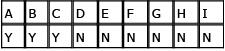Out of our 9 total places, the first three, A, B, and C, win medals, so we label these with a "Y." The final six places (D, E, F, G, H, and I) do not win medals, so we label these with an "N." We translate this into math: 9! / 3!6! = 84. So our total possible number of combinations is 84. (Remember that ! means factorial; for example, 6! = 6 × 5 × 4 × 3 × 2 × 1.)
Note that although the problem seemed to make a point of differentiating the first, second, and third places, our question asks only whether the brothers will medal, not which place they will win. This is why we don't need to worry about labeling first, second, and third place distinctly.
Now, we need to determine the number of instances when at least two brothers win a medal. Practically speaking, this means we want to add the number of instances two brothers win to the number of instances three brothers win.
Let's start with all three brothers winning medals, where B represents a brother.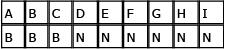Since all the brothers win medals, we can ignore the part of the counting grid that includes those who don't win medals. We have 3! / 3! = 1. That is, there is only one instance when all three brothers win medals.
Next, let's calculate the instances when exactly two brothers win medals.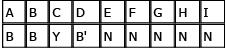Since brothers both win and don't win medals in this scenario, we need to consider both sides of the grid (i.e. the ABC side and the DEFGHI side). First, for the three who win medals, we have 3! / 2! = 3. For the six who don't win medals, we have 6! / 5! = 6. We multiply these two numbers to get our total number: 3 × 6 = 18.
Another way to consider the instances of at least two brothers medaling would be to think of simple combinations with restrictions. If you are choosing 3 people out of 9 to be winners, how many different ways are there to chose a specific set of 3 from the 9 (i.e. all the brothers)? Just one. Therefore, there is only one scenario of all three brothers medaling.
If you are choosing 3 people out of 9 to be winners, if 2 specific people of the 9 have to be a member of the winning group, how many possible groups are there? It is best to think of this as a problem of choosing 1 out of 7 (2 must be chosen). Choosing 1 out of 7 can be represented as 7! / 1!6! = 7. However, if 1 of the remaining 7 can not be a member of this group (in this case the 3rd brother) there are actually only 6 such scenarios. Since there are 3 different sets of exactly two brothers (B1B2, B1B3, B2B3), we would have to multiply this 6 by 3 to get 18 scenarios of only two brothers medaling. The brothers win at least two medals in 18 + 1 = 19 circumstances. Our total number of circumstances is 84, so our probability is 19 / 84.

QUESTION: 7

Set S is the set of all prime integers between 0 and 20. If three numbers are chosen randomly from set S and each number can be chosen only once, what is the positive difference between the probability that the product of these three numbers is a number less than 31 and the probability that the sum of these three numbers is odd?

Solution:

If set S is the set of all prime integers between 0 and 20 then: S = {2, 3, 5, 7, 11, 13, 17, 19}
Let’s start by finding the probability that the product of the three numbers chosenis a number less than 31. To keep the product less than 31, the three numbers must be 2, 3 and 5. So, what is the probability that the three numbers chosen will be some combination of 2, 3, and 5?
Here’s the list all possible combinations of 2, 3, and 5: case A: 2, 3, 5 case B: 2, 5, 3 case C: 3, 2, 5 case D: 3, 5, 2 case E: 5, 2, 3 case F: 5, 3, 2 This makes it easy to see that when 2 is chosen first, there are two possible combinations. The same is true when 3 and 5 are chosen first. The probability of drawing a 2, AND a 3, AND a 5 in case A is calculated as follows (remember, when calculating probabilities, AND means multiply): case A: (1/8) x (1/7) x (1/6) = 1/336 The same holds for the rest of the cases. case B: (1/8) x (1/7) x (1/6) = 1/336 case C: (1/8) x (1/7) x (1/6) = 1/336 case D: (1/8) x (1/7) x (1/6) = 1/336 case E: (1/8) x (1/7) x (1/6) = 1/336 case F: (1/8) x (1/7) x (1/6) = 1/336 So, a 2, 3, and 5 could be chosen according to case A, OR case B, OR, case C, etc. The total probability of getting a 2, 3, and 5, in any order, can be calculated as follows (remember, when calculating probabilities, OR means add): (1/336) + (1/336) + (1/336) + (1/336) + (1/336) + (1/336) = 6/336
Now, let’s calculate the probability that the sum of the three numbers is odd. In order to get an odd sum in this case, 2 must NOT be one of the numbers chosen. Using the rules of odds and evens, we can see that having a 2 would give the following scenario: even + odd + odd = even So, what is the probability that the three numbers chosen are all odd? We would need an odd AND another odd, AND another odd: (7/8) x (6/7) x (5/6) = 210/336 The positive difference between the two probabilities is: (210/336) – (6/336) = (204/336) = 17/28

QUESTION: 8

A random 10-letter code is to be formed using the letters A, B, C, D, E, F, G, H, I and I (only the “I” will be used twice). What is the probability that a code that has the two I’s adjacent to one another will be formed?

Solution:

To find the probability of forming a code with two adjacent I’s, we must find the total number of such codes and divide by the total number of possible 10-letter codes. The total number of possible 10-letter codes is equal to the total number of anagrams that can be formed using the letters ABCDEFGHII, that is 10!/2! (we divide by 2! to account for repetition of the I's).
To find the total number of 10 letter codes with two adjacent I’s, we can consider the two I’s as ONE LETTER. The reason for this is that for any given code with adjacent I’s, wherever one I is positioned, the other one must be positioned immediately next to it. For all intents and purposes, we can think of the 10 letter codes as having 9 letters (I-I is one). There are 9! ways to position 9 letters.
Probability = (# of adjacent I codes) / (# of total possible codes) = 9! ÷ (10! / 2! ) = ( 9!2! / 10! ) = (9!2! / 10(9!) ) = 1/5

QUESTION: 9

If p2 – 13p + 40 = q , and p is a positive integer between 1 and 10, inclusive, what is the probability that   q < 0?

Solution:

If we factor the right side of the equation, we can come up with a more meaningful relationship between p and q : p2 – 13p + 40 = q so (p – 8)(p – 5) = q. We know that p is an integer between 1 and 10, inclusive, so there are ten possible values for p. We see from the factored equation that the sign of q will depend on the value of p . One way to solve this problem would be to check each possible value of p to see whether it yields a positive or negative q. However, we can also use some logic here. For q to be negative, the expressions (p – 8) and (p – 5) must have opposite signs. Which integers on the number line will yield opposite signs for the expressions (p – 8) and (p – 5)? Those integers in the range 5 < p < 8 (notice 5 and 8 are not included because they would both yield a value of zero and zero is a nonnegative integer). That means that there are only two integer values for p, 6 and 7, that would yield a negative q. With a total of 10 possible p values, only 2 yield a negative q, so the probability is 2/10 or 1/5.

QUESTION: 10

A box cont ains three pairs of blue gloves and two pairs of green gloves. Each pair consists of a left-hand glove and a right-hand glove. Each of the gloves is separate from its mate and thoroughly mixed together with the others in the box. If three gloves are randomly selected from the box, what is the probability that a matched set (i.e., a left- and right-hand glove of the same color) will be among the three gloves selected?

Solution:

The simplest way to approach a complex probability problem is not always the direct way. In order to solve this problem directly, we would have to calculate the probabilities of all the different ways we could get two oppositehanded, same-colored gloves in three picks. A considerably less taxing approach is to calculate the probability of NOT getting two such gloves and subtracting that number from 1 (remember that the probability of an event occurring plus the probability of it NOT occurring must equal 1).
Let's start with an assumption that the first glove we pick is blue. The hand of the first glove is not important; it could be either right or left. So our first pick is any blue. Since there are 3 pairs of blue gloves and 10 gloves total, the probability of selecting a blue glove first is 6/10.
Let's say our second pick is the same hand in blue. Since there are now 2 blue gloves of the same hand out of the 9 remaining gloves, the probability of selecting such a glove is 2/9.
Our third pick could either be the same hand in blue again or any green. Since there is now 1 blue glove of the same hand and 4 green gloves among the 8 remaining gloves, the probability of such a pick is (1 + 4)/8 or 5/8. The total probability for this scenario is the product of these three individual probabilities: 6/10 x 2/9 x 5/8 = 60/720.
We can summarize this in a chart: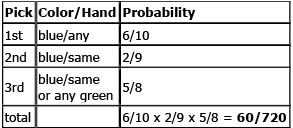We can apply the same principles to our second scenario, in which we choose blue first, then any green, then either the same-handed green or the same-handed blue: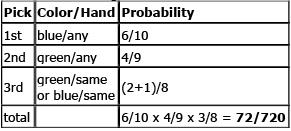But it is also possible to pick green first. We could pick any green, then the same-handed green, then any blue: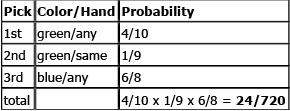Or we could pick any green, then any blue, then the same-handed green or same-handed blue: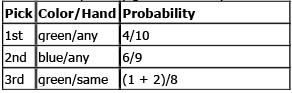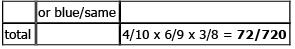The overall probability of NOT getting two gloves of the same color and same hand is the SUM of the probabilities of these four scenarios: 60/720 + 72/720 + 24/720 + 72/720 = 228/720 = 19/60.
Therefore, the probability of getting two gloves of the same color and same hand is 1 - 19/60 = 41/60.

QUESTION: 11

A football team has 99 players. Each pl ayer has a uniform number from 1 to 99 and no two players share the same number. When football practice ends, all the players run off the field one-by-one in a completely random manner. What is the probability that the first four players off the field will leave in order of increasing uniform numbers (e.g., #2, then #6, then #67, then #72, etc)?

Solution:

Every player has an equal chance of leaving at any particular time. Thus, the probability that four particular players leave the field first is equal to the probability that any other four players leave the field first. In other words, the answer to this problem is completely independent of which four players leave first.
Given the four players that leave first, there are 4! or 24 orders in which these players can leave the field - only one of which is in increasing order of uniform numbers. (For example, assume the players have the numbers 1, 2, 3, and 4. There are 24 ways to arrange these 4 numbers: 1234, 1243, 1324, 1342, 1423, 1432, . . . , etc. Only one of these arrangements is in increasing order.)
Thus, the probability that the first four players leave the field in increasing order of their uniform numbers is 1/24.

QUESTION: 12

Bill and Jane pl ay a simple game involving two fair dice, each of which has six sides numbered from 1 to 6 (with an equal chance of landing on any side). Bill rolls the dice and his score is the total of the two dice. Jane then rolls the dice and her score is the total of her two dice. If Jane’s score is higher than Bill’s, she wins the game. What is the probability the Jane will win the game?

Solution:

Since each die has 6 possible outcomes, there are 6 × 6 = 36 different ways that Bill can roll two dice. Similarly there are 6 × 6 = 36 different ways for Jane to roll the dice. Hence, there are a total of 36 × 36 = 1296 different possible ways the game can be played.
One way to approach this problem (the hard way) is to consider, in turn, the number of ways that Bill can get each possible score, compute the number of ways that Jane can beat him for each score, and then divide by 1296. The number of ways to make each score is: 1 way to make a 2 (1 and 1), 2 ways to make a 3 (1 and 2, or 2 and 1), 3 ways to make a 4 (1 and 3, 2 and 2, 3 and 1), 4 ways to make a 5 (use similar reasoning…), 5 ways to make a 6, 6 ways to make a 7, 5 ways to make an 8, 4 ways to make a 9, 3 ways to make a 10, 2 ways to make an 11, and 1 way to make a 12. We can see that there is only 1 way for Bill to score a 2 (1 and 1). Since there are 36 total ways to roll two dice, there are 35 ways for Jane to beat Bob's 2.
Next, there are 2 ways that Bob can score a 3 (1 and 2, 2 and 1). There are only three ways in which Jane would not beat Bob: if she scores a 2 (1 and 1), she would lose to Bob or if she scores a 3 (1 and 2, 2 and 1), she would tie Bob. Since there are 36 total ways to roll the dice, Jane has 33 ways to beat Bob.
Using similar logic, we can quickly create the following table: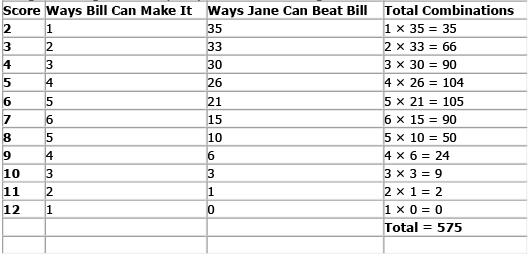Out of the 1296 possible ways the game can be played, 575 of them result in Jane winning the game. Hence, the probability the Jane will win is 575/1296 and the correct answer is C.
There is a much easier way to compute this probability. Observe that this is a “symmetric” game in that neither Bill nor Jane has an advantage over the other. That is, each has an equal change of winning. Hence, we can determine the number of ways each can win by subtracting out the ways they can tie and then dividing the remaining possibilities by 2.
Note that for each score, the number of ways that Jane will tie Bill is equal to the number of ways that Bill can make that score (i.e., both have an equal number of ways to make a particular score). Thus, referring again to the table above, the total number of ways to ti e are: 12 + 22 + 32 + 42 +52 +62 + 52 + 42 + 32 + 22 + 12 = 146. Therefore, there are 1296 – 146 = 1150 non-ties. Since this is a symmetric problem, Jane will win 1150/2 or 575 times out of the 1296 possible games. Hence, the probability that she will win is 575/1296.

QUESTION: 13

Kate and Danny each have \$10. Together, they flip a fair coin 5 times. Every time the coin lands on heads, Kate gives Danny \$1. Every time the coin lands on tails, Danny gives Kate \$1. After the five coin flips, what is the probability that Kate has more than \$10 but less than \$15?

Solution:

Let's consider the different scenarios: If Kate wins all five flips, she ends up with \$15.
If Kate wins four flips, and Danny wins one flip, Kate is left with \$13.
If Kate wins three flips, and Danny wins two flips, Kate is left with \$11.
If Kate wins two flips, and Danny wins three flips, Kate is left with \$9.
If Kate wins one flip, and Danny wins four flips, Kate is left with \$7.
If Kate loses all five flips, she ends up with \$5.
The question asks for the probability that Kate will end up with more than \$10 but less than \$15. In other words, we need to determine the probability that Kate is left with \$11 or \$13 (since there is no way Kate can end up with \$12 or \$14).
The probability that Kate ends up with \$11 after the five flips:
Since there are 2 possible outcomes on each flip, and there are 5 flips, the total number of possible outcomes is 2*2*2*2*2=32. Thus, the five flips of the coin yield 32 different outcomes.
To determine the probability that Kate will end up with \$11, we need to determine how many of these 32 outcomes include a combination of exactly three winning flips for Kate.
We can create a systematic list of combinations that include three wins for Kate and two wins for Danny: DKKKD, DKKDK, DKDKK, DDKKK, KDKKD, KDKDK, KDDKK, KKDKD, KKDDK, KKKDD = 10 ways.
Alternatively, we can consider each of the five flips as five spots. There are 5 potential spots for Kate's first win. There are 4 potential spots for Kate's second win (because one spot has already been taken by Kate's first win). There are 3 potential spots for Kate's third win. Thus, there are 5*4*3=60 ways for Kate's three victories to be ordered. However, since we are interested only in unique winning combinations, this number must be reduced due to overcounting. Consider the winning combination KKKDD: This one winning combination has actually been counted 6 times (this is 3! or three factorial) because there are 6 different orderings of this one combination: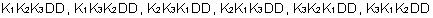This overcounting by 6 is true for all of Kate's three-victory combinations. Therefore, there are only 60/6 =10, ways for Kate to have three wins and end up with \$11 (as we had discovered earlier from our systematic list).
The probability that Kate ends up with \$13 after the five flips: To determine the probability that Kate will end up with \$13, we need to determine how many of the 32 total possible outcomes include a combination of exactly four winning flips for Kate.
Again, we can create a systematic list of combinations that include four wins for Kate and one win for Danny: KKKKD, KKKDK, KKDKK, KDKKK, DKKKK = 5 ways.
Alternatively, using the same reasoning as above, we can determine that there are 5*4*3*2 = 120 ays for Kate's four victories to be ordered. Then, reduce this by 4! (four factorial) or 24 due to overcounting. Thus, there are 120/24 = 5ways for Kate to have four wins and end up with \$13 (the same answer we found using the systematic list).
The total probability that Kate ends up with either \$11 or \$13 after the five flips: There are 10 ways that Kate is left with \$11. There are 5 ways that Kate is left with \$13. Therefore, there are 15 ways that Kate is left with more than \$10 but less than \$15.
Since there are 32 possible outcomes, is 15/32.

QUESTION: 14

There is a 10% chance that it won’t snow all winter long. There is a 20% chance that schools will not be closed all winter long. What is the greatest possible probability that it will snow and schools will be closed during the winter?

Solution:

There is a strong temptation to solve this problem by simply finding the probability that it will snow (90%) and the probability that schools will be closed (80%) and multiplying these two probabilties. This approach would yield the incorrect answer (72%), choice D. However, it is only possible to multiply probabilities of separate events if you know that they are independent from each other. This fact is not provided in the problem. In fact, we would assume that school being closed and snow are, at least to some extent, dependent on each other. However, they are not entirely dependent on each other; it is possible for either one to happen without the other. Therefore, there is an unknown degree of dependence; hence there is a range of possible probabilities, depending on to what extent the events are dependent on each other. Set up a matrix as shown below. Fill in the probability that schools will not be closed and the probability that there will be no snow.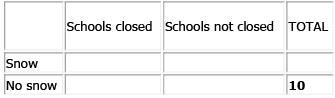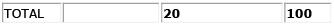Then use subtraction to fill in the probability that schools will be closed and the probability that there will be snow.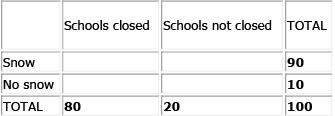To find the greatest possible probability that schools will be closed and it will snow, fill in the remaining cells with the largest possible number in the upper left cell.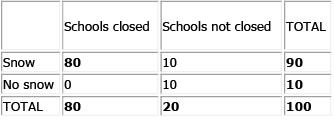The greatest possible probability that schools will be closed and it will snow is 80%.

QUESTION: 15

There are y different travelers who each have a choice of vacationing at one of n different destinations. What is the probability that all y travelers will end up vacationing at the same destination?

Solution:

The easiest way to attack this problem is to pick some real, easy numbers as values for y and n . Let's assume there are 3 travelers (A, B, C) and 2 different destinations (1, 2). We can chart out the possibilities as follows: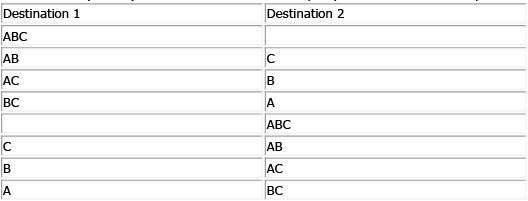Thus there are 8 possibilities and in 2 of them all travelers end up at the same destination. Thus the probability is 2/8 or 1/4. By plugging in y = 3 and n = 2 into each answer choice, we see that only answer choice D yields a probability of 1/4. Alternatively, consider that each traveler can end up at any one of n destinations. Thus, for each traveler there are n possibilities. Therefore, for y travelers, there are possible outcomes. Additionally, the "winning" outcomes are those where all travelers end up at the same destination. Since there are n destinations there are n "winning" outcomes.
Thus, the probability =